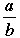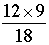SEARCH HOMEMath Central Quandaries & QueriesQuestion from shradheya, a student: write all fall using variable ,number, and basic operation 1. divide the product of 'x' and '3y' by '2z'.Hi,

Try this. Using the notation × for multiplication and the notationfor division I would respond to "divide the product of '12' and '9' by '18'" byUsing the same notation for the mathematical operations write a response to "divide the product of 'x' and '3y' by '2z'".

PennyMath Central is supported by the University of Regina and The Pacific Institute for the Mathematical Sciences.